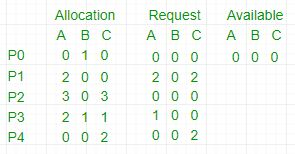# Deadlock Detection Algorithm in Operating System

If a system does not employ either a deadlock prevention or deadlock avoidance algorithm then a deadlock situation may occur. In this case-

• Apply an algorithm to examine state of system to determine whether deadlock has has occurred or not.
• Apply an algorithm to recover from the deadlock. For more refer- Deadlock Recovery

The algorithm employs several time varying data structures:

• Available- A vector of length m indicates the number of available resources of each type.
• Allocation- An n*m matrix defines the number of resources of each type currently allocated to a process. Column represents resource and resource represent process.
• Request- An n*m matrix indicates the current request of each process. If request[i][j] equals k then process Pi is requesting k more instances of resource type Rj.

We treat rows in the matrices Allocation and Request as vectors, we refer them as Allocationi and Requesti.

Steps of Algorithm:

1. Let Work and Finish be vectors of length m and n respectively. Initialize Work= Available. For i=0, 1, …., n-1, if Requesti = 0, then Finish[i] = true; otherwise, Finish[i]= false.
2. Find an index i such that both
a) Finish[i] == false
b) Requesti <= Work
If no such i exists go to step 4.
3. Work= Work+ Allocationi
Finish[i]= true
Go to Step 2.
4. If Finish[i]== false for some i, 0<=i<n, then the system is in a deadlocked state. Moreover, if Finish[i]==false the process Pi is deadlocked.

For example,1. In this, Work = [0, 0, 0] &
Finish = [false, false, false, false, false]
2. i=0 is selected as both Finish = false and [0, 0, 0]<=[0, 0, 0].
3. Work =[0, 0, 0]+[0, 1, 0] =>[0, 1, 0] &
Finish = [true, false, false, false, false].
4. i=2 is selected as both Finish = false and [0, 0, 0]<=[0, 1, 0].
5. Work =[0, 1, 0]+[3, 0, 3] =>[3, 1, 3] &
Finish = [true, false, true, false, false].
6. i=1 is selected as both Finish = false and [2, 0, 2]<=[3, 1, 3].
7. Work =[3, 1, 3]+[2, 0, 2] =>[5, 1, 5] &
Finish = [true, true, true, false, false].
8. i=3 is selected as both Finish = false and [1, 0, 0]<=[5, 1, 5].
9. Work =[5, 1, 5]+[2, 1, 1] =>[7, 2, 6] &
Finish = [true, true, true, true, false].
10. i=4 is selected as both Finish = false and [0, 0, 2]<=[7, 2, 6].
11. Work =[7, 2, 6]+[0, 0, 2] =>[7, 2, 8] &
Finish = [true, true, true, true, true].
12. Since Finish is a vector of all true it means there is no deadlock in this example.My Personal Notes arrow_drop_upStudent at Netaji Subhas Institute of Technology

If you like GeeksforGeeks and would like to contribute, you can also write an article using contribute.geeksforgeeks.org or mail your article to contribute@geeksforgeeks.org. See your article appearing on the GeeksforGeeks main page and help other Geeks.

Please Improve this article if you find anything incorrect by clicking on the "Improve Article" button below.

Article Tags :
Practice Tags :

3

Please write to us at contribute@geeksforgeeks.org to report any issue with the above content.